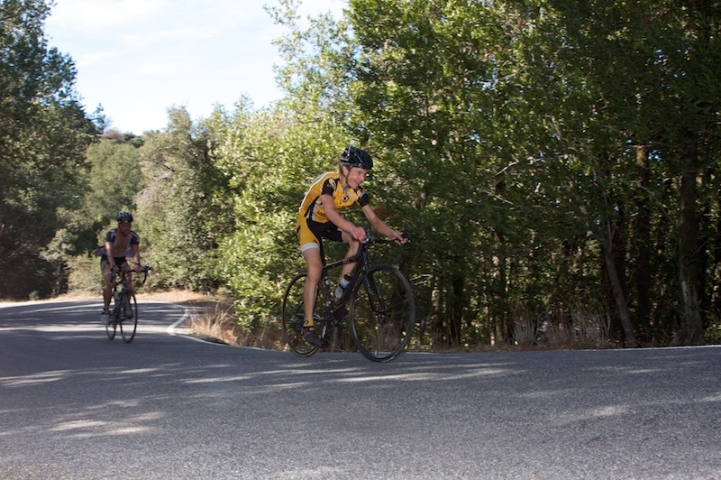## Tuesday, October 27, 2009

### Soda Springs power: error estimate

Last post I made an estimate of my power up Soda Springs road on Saturday. It's worth doing an estimate of possible errors with this:
• body mass: around 1 lb
• equipment mass: let's say 1 lb
• rolling resistance coefficient: 20% seems reasonable. This was really seat-of-the-pants.
• wind drag coefficient: 10% seems about right
• distance 0.1 miles. Exact starting location, the placement of the finish, and line through the corners are all factors
• altitude gained: 15 feet sees consistent with individual reports
• wind speed: Zero was assumed. Given variable winds reported at Redwood Estates, a conservative estimate of error of this estimate is probably 1 mph
• transmission coefficient: based on differences in chainline, I'll say 1%. This doesn't affect the derived Powertap-equivalend power, but it does affect the significance of comparisons in power from one ride to the next.
• time: 2 seconds seems good: 1 second with recording the finish time, and 1 second for a delay in starting from when the horn was honked
• acceleration: I may have crossed the finish as fast as 15 mph
Let's run the numbers, then, on how each of these errors would affect the result. Fearlessly diving in:
• body mass & equipment mass: I'm assuming each affects power the same. These affect climbing power, rolling resistance power, and acceleration power. The resulting changes are:
1. climbing power: ΔP = 1.7 W
2. rolling resistance watts: ΔP = 0.08 W
3. acceleration power: ΔP = 0.02 W.
• rolling resistance coefficient: changes rolling resistance power in proportion to error: ΔP = 2.2 W.
• wind resistance coefficient: changes wind resistance power in proportion to error: ΔP = 1.8 W
• distance: this affects wind resistance, rolling resistance, and acceleration power. First, a 0.1 mile difference out of 5.35 miles is a 1.87% difference in speed. From this:
1. Wind resistance increases by approximately 3 × 1.87%, +5.71%: ΔP = 1.0 W.
2. rolling resistance increases by 1.87%: ΔP = 0.21 W.
3. Acceleration power increases by approximately 2 × 1.87%, ΔP = 0.01 W
• altitude gained: weight × height / time: ΔP = 1.5 W
• wind speed: wind resistance changed proportional to twice the ratio of wind speed error to bike speed: ΔP = 3.6 W.
• transmission coefficient: error in power = error in transmission coefficient × total power: ΔP = 2.6 W
• time: the time error was 0.10%. This affects climbing and rolling resistance power by the same fraction. Wind resistance power is affected by 3 times this proportion. Acceleration power is affected by twice this proportion (an insignificant contribution). In total: ΔP = 0.31 W
• acceleration: changes acceleration power by up to +125%: ΔP = 0.4 W
Whew! Okay, so you might be tempted to add up all of these error terms as "worst case" estimation (in circuit design this is called "designing to the worst-case corners"), but for uncorrelated errors (for example, an error in distance doesn't imply anything about the error in time or weight) the better approach is to sum the squares of the error terms, then take the square root.Josh Hadley photo

Okay.... drum roll....
ΔP = 6.1 W

So my estimate of 263 W could in effect be anywhere from 257 W up to 269 W. Given the 8 W difference from OLH distance to Soda Springs distance, that would be an effective OLH power of as high as 277 W powertap-equivalent. Were I to have extracted that value, given my present fitness, I'd not be concerned, especially since there's similar error bars on that 290 W estimate from my Old La Honda climb this summer.

So the end result is: don't place too much importance in these sorts of physics-based power estimates. There's too many variables, too many sources of error, even for something as straightforward as a steep hillclimb.

Perhaps surprising in the strong influence of wind resistance on the error estimate: that term alone increased the uncertainty by 1.2 W. And we made a seemingly trivial estimate of the uncertainty in the wind speed: only 1 mph, essentially impossible to detect without instruments.

So don't sell that power meter yet.

phipps said...

Dan, thanks for that clarification!

So, could someone else estimate their wattage by using the following equation?:

djconnel said...

Not quite....

The issue is the wind resistance power isn't proportional to speed. You can apply that equation to the climbing power, and to the rolling resistance power times the ratio of rolling resistances (with clinchers and butyl tubes at less than my 140 psi you were probably 20% higher rolling resistance in addition to the mass ratio). But for the wind resistance you should multiply by the cube of the speed and by an additional factor proportional to the cross-sectional area (which is roughly the mass to the 3/4 power, to decent approximation).

So your power would be, where

Pc0 = my climbing power
Pr0 = my rolling power
Pw0 = my wind power
Mb0 = my body mass
Me0 = my equipment + bike mass
Crr0 = my rolling resistance
coefficient
v0 = my speed